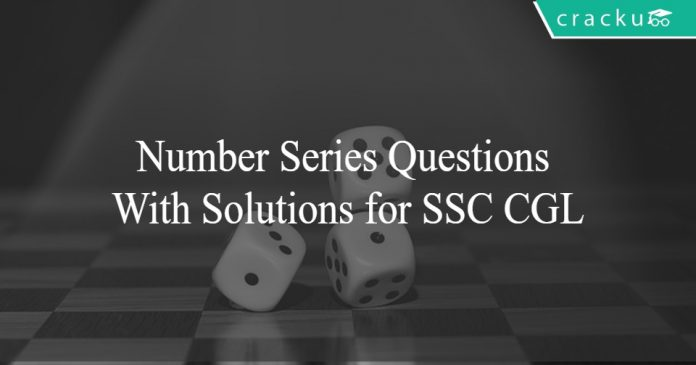# Number Series Questions With Solutions for SSC CGL PDF

1
5768Number Series Questions With Solutions for SSC CGL

Number Series Questions With Solutions for SSC CGL PDF:

Number series questions and answers with explanation for SSC CGL exam. Detailed solutions of number series problems with solving tricks.

Question 1: Which number is wrong in the given series?
12439, 23549, 34659, 45769

a) 34659
b) 23549
c) 45769
d) 12439

Question 2: Find the missing number :
2, 10, 20, 32, 46, ?

a) 62
b) 65
c) 64
d) 60

Question 3: What will come in place of the question mark (?) in the series?
3, 8, 27, 112, (?), 3396

a) 565
b) 452
c) 560
d) 678

Question 4: If 1 + 4=9, 2 + 8=18, 3+6 = 15 then 7 + 8 = ?

a) 41
b) 23
c) 30
d) 32

Question 5: Find the wrong number in the series. 6, 9, 15, 22, 51, 99

a) 99
b) 51
c) 22
d) 15

Question 6: Find out the set of numbers amongst the four sets of numbers given in the alternatives which is most like the set given in the question.
Given Set : (8, 56, 72)

a) (7, 56, 63)
b) (3, 15, 24)
c) (6. 42, 54)
d) (5, 30, 35)

Question 7: Find the wrong number in the series. 7, 28, 63,124, 215, 342

a) 28
b) 63
c) 124
d) 342

Question 8: Find the correct set of numbers from the given alternatives.
(4, 3, 2), (16, 9, 4), (256, 81, 16), (65536, ? , ? )

a) 6651, 286
b) 6561, 256
c) 1486, 97
d) 190, 20

Question 9: Which of the given letter groups follows the same principle on which the numbers are arranged in the given number group ?
(5, 8, 13, 20, 29)

a) ACGKR
b) ADIMS
c) ADIPY
d) ADGRO

Question 10: Find out the wrong number in the sequence
102, 101, 98, 93, 86, 74, 66, 53

a) 101
b) 66
c) 74
d) 93

Solutions for Number Series Questions With Solutions for SSC CGL PDF:

Solutions:

1) Answer (A)

here we need to find the wrong number in 12439, 23549, 34659, 45769
as we will add the digits of every number
12439 : 1+2+3+4+9 = 19
23549 : 2+3+5+4+9 = 23
34659 : 3+4+6+5+9 = 27
45769 : 4+5+7+6+9 = 31
As we can see that in very case the sum of digits of number is a prime number except in case of 34659 and hence 34659 is the odd one out

2) Answer (A)

let the missing number be y
10 – 2 = 8
20 – 10 = 10
32 – 20 = 12
46 – 32 = 14
as we can see that difference is getting increased by 2 and hence
y – 46 = 16
y = 62

3) Answer (A)

Let the missing term be y
here the sequence is 3, 8, 27, 112, y, 3396
here the pattern is :
8 = 3 x 2 + 2
27 = 8 x 3 + 3
112 = 27 x 4 + 4
so from the highlighted pattern , we can say that
y = 112 x 5 + 5 = 565

4) Answer (B)

Here we are given :
1 + 4=9
2 + 8=18
3+6 = 15
So if the number is , x + y then it is equal to [(x+y) + y]
here we need to calculate value of 7 + 8 = [(7 + 8) + 8] = 23

5) Answer (C)

9 -6 =3
15 -9 =6
22 -15 =7
51 -22 =29
99 -51 =48
So Ignoring the difference between the terms where one of the terms is 22 all other differences are multiple of 3
So 22 is the wrong term and the correct series would have been
9 -6 = 3 x 1
15 -9 = 3 x 2
27 -15 = 3 x 4
51 -27 = 3 x 8
99 -51 = 3 x 16
So 22 is the wrong term

6) Answer (C)

(8, 56, 72)
follows the pattern
(8 , 8 x 7 , 8 x 9 )
So (6. 42, 54) is similar to (8, 56, 72)
as it follows the pattern
(6 , 6 x 7 , 6 x 9 )

7) Answer (A)

By observing the terms of series it can be deduced that the series must follow the pattern
$$2^3 -1$$,$$3^3 -1$$,$$4^3 -1$$,$$5^3 -1$$,$$6^3 -1$$,$$7^3 -1$$
So option A is correct

8) Answer (B)

In the given series each element of the next term is obtained by squaring the corresponding element of each previous term
So
the missing second and third value from the last term is
$$81^{2}$$ =6561 and
$$16^{2}$$ = 256
respectively

9) Answer (C)

The Series follows the pattern
5
5+3 =8
8+5 =13
13 +7 =20
20+9 = 29
So the option representing the correct letter group must follow the same pattern
So
the letter series is
A
A+3 = D
D +5 =I
I +7 = P
P + 9 =Y
So the letter series is ADIPY

10) Answer (C)

The Series follows pattern
102
102 -1 =101
101 -3 =98
98 -5 =93
93 -7 =86
Since 1,3,5 and 7 are subtracted in order to get the next term
So 7 +2 =9
should be subtracted in order to get next term
So
next term should be
86 -9 =77
77 -11 =66
66-13 =53
So option C is correct

Practice this SSC CGL Number series questions with answers to understand and prepare for the upcoming exam.